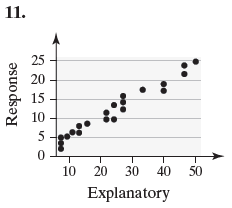×
Get Full Access to Statistics: Informed Decisions Using Data - 5 Edition - Chapter 4.1 - Problem 11
Get Full Access to Statistics: Informed Decisions Using Data - 5 Edition - Chapter 4.1 - Problem 11

×

# ?In Problems 9–12, determine whether the scatter diagram indicates that a linear relation may exist between the two variables. If the relation is lineaISBN: 9780134133539 240

## Solution for problem 11 Chapter 4.1

Statistics: Informed Decisions Using Data | 5th Edition

• Textbook Solutions
• 2901 Step-by-step solutions solved by professors and subject experts
• Get 24/7 help from StudySoup virtual teaching assistantsStatistics: Informed Decisions Using Data | 5th Edition

4 5 1 263 Reviews
24
1
Problem 11

In Problems 9–12, determine whether the scatter diagram indicates that a linear relation may exist between the two variables. If the relation is linear, determine whether it indicates a positive or negative association between the variables.Step-by-Step Solution:

Step 1 of 5) In Problems 9–12, determine whether the scatter diagram indicates that a linear relationship may exist between the two variables. If the relation is linear, determine whether it indicates a positive or negative association between the variables. Approach We will use StatCrunch to construct the frequency and relative frequency histograms. The steps for constructing the graphs using the TI-83/84 Plus graphing calculators, Minitab, Excel, and StatCrunch, are given in the Technology Step-by-Step on pages 86–87. Solution Figures 9(a) and (b) show the frequency and relative frequency histograms, respectively, obtained from StatCrunch.

Step 2 of 2

##### ISBN: 9780134133539

This textbook survival guide was created for the textbook: Statistics: Informed Decisions Using Data, edition: 5. This full solution covers the following key subjects: . This expansive textbook survival guide covers 88 chapters, and 2422 solutions. Since the solution to 11 from 4.1 chapter was answered, more than 210 students have viewed the full step-by-step answer. The answer to “?In 9–12, determine whether the scatter diagram indicates that a linear relation may exist between the two variables. If the relation is linear, determine whether it indicates a positive or negative association between the variables.” is broken down into a number of easy to follow steps, and 35 words. Statistics: Informed Decisions Using Data was written by and is associated to the ISBN: 9780134133539. The full step-by-step solution to problem: 11 from chapter: 4.1 was answered by , our top Statistics solution expert on 01/15/18, 03:19PM.

## Discover and learn what students are asking

Calculus: Early Transcendental Functions : Functions of Several Variables
?In Exercises 15 - 22, find all first partial derivatives. $$f(x, y)=4 x^{2}-2 x y+y^{2}$$

Calculus: Early Transcendental Functions : Introduction to Functions of Several Variables
?In Exercises 7-18, find and simplify the function values. $$f(x, y)=3 x^{2}-2 y$$ (a) $$\frac{f(x+\Delta x, y)-f(x, y)}{\Delta x}$$

Statistics: Informed Decisions Using Data : Testing the Significance of the Least-Squares Regression Model
?In Problems 5–10, use the results of Problems 7–12, respectively, from Section 4.2 to answer the following questions: (a) What are the estima

Statistics: Informed Decisions Using Data : Inference about the Difference between Two Medians: Independent Samples
?In Problems 1–8, use the Mann–Whitney test to test the given hypotheses at the = 0.05 level of significance. The independent samples were obtained ra

Chemistry: The Central Science : The Chemistry of Life: Organic and Biological Chemistry
?Identify the carbon atom(s) in the structure shown that has (have) each of the following hybridizations: (a) s p^{3}, (b) sp,

Unlock Textbook Solution